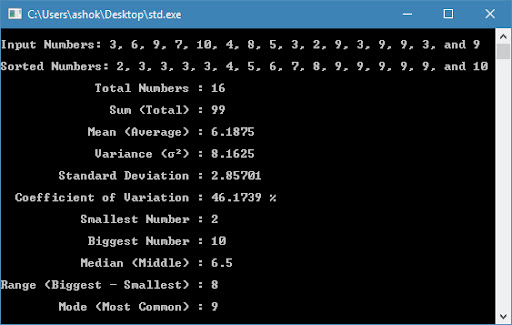## Posts

Showing posts from July, 2018

### Computing Sum, Mean, Variance, Standard Deviation, Coefficient of Variation, Smallest, Biggest, Median, Range, and Mode using C++This is a programming tutorial for computing sum, mean, variance, standard deviation, coefficient of variation, smallest number, biggest number, median, range, and mode of n numbers using C++. In this tutorial, I have used Dev C++ v5.11 software for compiling the C++ program. The formula for calculating sum, mean, variance, standard deviation, coefficient of variation, smallest number, biggest number, median, range, and mode of set of n numbers is given below: Description Formula Number of Samples n Sum (Total) $\sum\limits_{i=1}^{n}{{{x}_{i}}}={{x}_{1}}+{{x}_{2}}+...+{{x}_{n}}$ Mean (Average) $\bar{x}=\frac{\sum\limits_{i=1}^{n}{{{x}_{i}}}}{n}$ Variance ${{\operatorname{var}}_{x}}=\frac{\sum\limits_{i=1}^{n}{{{({{x}_{i}}-\bar{x})}^{2}}}}{n-1}$ Standard Deviation ${{\sigma }_{x}}=\sqrt{\frac{\sum\limits_{i=1}^{n}{{{({{x}_{i}}-\bar{x})}^{2}}}}{n-1}}$ Coefficient of